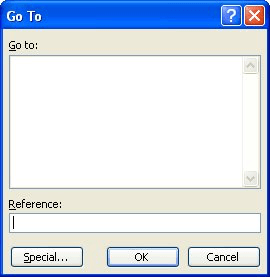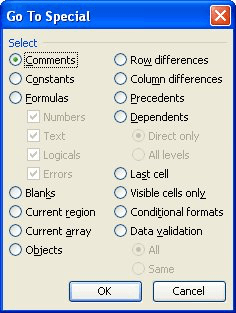# Returning a Blank Valueby Allen Wyatt
(last updated November 21, 2020)

The purpose of formulas is to return a value based upon a calculation or comparison. For instance, if you use the formula =1+1, the sum is calculated and the value 2 is returned. There might be times, however, when you want to use a formula and actually return nothing. For instance, you might want to compare a value in a cell to some constant and return a numeric value if they are equal or return nothing if they are different.

The problem is that a formula must, under all circumstances, return something—for it not to do so would go against the very purpose of formulas. There are two ways to approach the problem, and how you do so will affect what you can and cannot do with your results. Consider the following formula:

```=IF(A1=0,"",1)
```

In this instance, the cell containing this will contain a blank value ("") if A1 is 0 or it will contain a numeric value (1) if it is not 0. In either case, something is being returned. You could, however, use the following variation on the formula:

```=IF(A1=0,,1)
```

The only difference here, of course, is that the quote marks have been removed. Interestingly enough, in this case Excel assumes there is a zero between the two consecutive commas, and if A1 is 0, the formula returns a 0. Again, formulas must return something.

The way that Excel's other functions interpret the results of these two formulas is also very interesting. It is instructive to look at how the COUNT, COUNTA, and COUNTBLANK functions interpret the results.

COUNT is used to count the number of cells in a range that contain numeric values. If the cells contain text, or if they are empty, they are ignored. In the case of our formulas, if you use the first formula, COUNT counts the cell if A1 is not zero. If you use the second formula, COUNT will always count the result, since it always returns either 0 or 1, which are both numeric.

COUNTA is used to count the number of cells in a range that contain anything. Regardless of which formula you use, COUNTA will count the cell since formulas always return something. (It can also be argued that COUNTA counts the cell because it contains a formula, but that is probably a fine semantic difference.)

COUNTBLANK examines cells and counts them only if they are blank. In the case of the first formula, COUNTBLANK will count the cell only if A1 is 0. In the case of the second formula, COUNTBLANK will never count the cell, since the formula always returns a 0 or 1 and is therefore never blank.

The above discussion applies if the COUNT, COUNTA, or COUNTBLANK functions are evaluating the results of a series of cells that actually contain formulas. However, if the range includes cells that are really blank (i.e., they contain nothing, not even a formula), then that can affect what is returned by the functions. Blank cells don't affect the results returned by either COUNT or COUNTA, but they do affect the results returned by COUNTBLANK.

What does all this mean? It means that a cell that contains a formula is never really, truly blank—only cells with nothing in them are blank. How the result of the formula is interpreted, however, depends on the Excel functions being used to perform the interpretation. Since different functions interpret formula results differently, you need to be concerned with what you really want to find out about the formula results, and then use the function that will help you best determine that information. If you don't get the result you expect with a particular function, search around—chances are good that Excel has a different function you can use to get the desired results.

That being said, if you have a range of cells that all contain formulas similar to =IF(A1=0,"",1), and you want to delete the formulas in the cells that return a blank value (""), you can quickly do so by following these steps:

1. Select the range containing the formulas in question.
2. Press F5. Excel displays the Go To dialog box. (See Figure 1.)
3.Figure 1. The Go To dialog box.

4. Click Special. Excel displays the Go To Special dialog box. (See Figure 2.)
5.Figure 2. The Go To Special dialog box.

6. Make sure the Formulas radio button is selected.
7. The only check box that should be selected under Formulas is Text.
8. Click OK. Excel selects all cells where the formula returned a text value. (This should be all the formulas that returned "".)
9. Press Delete.

ExcelTips is your source for cost-effective Microsoft Excel training. This tip (2814) applies to Microsoft Excel 97, 2000, 2002, and 2003.

##### Author Bio

Allen Wyatt

With more than 50 non-fiction books and numerous magazine articles to his credit, Allen Wyatt is an internationally recognized author. He is president of Sharon Parq Associates, a computer and publishing services company. ...

##### MORE FROM ALLEN

Creating a Command List

Want a list of all the commands available in Word? You can get one easily by following these steps.

Discover More

Converting PDF to Excel

Reports and other formal documents are often distributed in PDF format so that they can be read and printed on a variety ...

Discover More

Excel can helpfully display some statistical information in the program's Status Bar. If you cannot see all the ...

Discover MoreProfessional Development Guidance! Four world-class developers offer start-to-finish guidance for building powerful, robust, and secure applications with Excel. The authors show how to consistently make the right design decisions and make the most of Excel's powerful features. Check out Professional Excel Development today!

Counting Displayed Cells

When you filter data, Excel displays only a portion of what is really in a worksheet. If you want to count the number of ...

Discover More

Working with Roman Numerals

Understanding and using a function to replace an Arabic number with Roman numerals. And, as a bonus, how to change them back.

Discover More

Calculating Fractions of Years

When working with dates and the relationship between dates, Excel provides a variety of worksheet functions that may ...

Discover More
##### Subscribe

FREE SERVICE: Get tips like this every week in ExcelTips, a free productivity newsletter. Enter your address and click "Subscribe."

If you would like to add an image to your comment (not an avatar, but an image to help in making the point of your comment), include the characters [{fig}] in your comment text. You’ll be prompted to upload your image when you submit the comment. Maximum image size is 6Mpixels. Images larger than 600px wide or 1000px tall will be reduced. Up to three images may be included in a comment. All images are subject to review. Commenting privileges may be curtailed if inappropriate images are posted.

What is 8 - 8?

2020-11-21 06:44:31

David D Delp

Could this be added to a macro that runs when cells are updated? Create a macro that first writes the formulas into the cells and then deletes the formulas based on the calculated values.

##### This Site

Got a version of Excel that uses the menu interface (Excel 97, Excel 2000, Excel 2002, or Excel 2003)? This site is for you! If you use a later version of Excel, visit our ExcelTips site focusing on the ribbon interface.